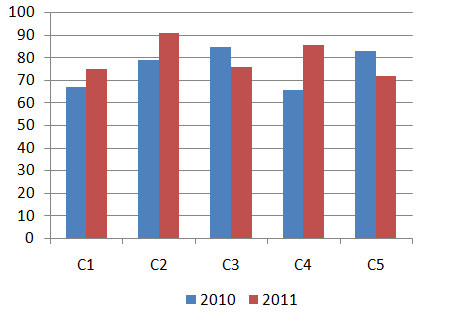# RPF 10 Jan 2019 Slot 1

Instructions

For the following questions answer them individually

Question 91

# Vimal obtained 46 marks out of 80 in French, 84 marks out of 100 in English, 44 out of 70 in Spanish and 36 out of 50 in Japanese. What is the overall percentage obtained by him? (in %)Question 92

# When a number divided by 119 it leaves a remainder 15. What will be the remainder, when the same number divided by 17.Question 93

# A sum of Rs.6800 invested under simple interest at rate of 8% p.a. If the amount after 5 years is been withdrawn and half of the total amount is been invested in Share market. Find the remaining amount. (in Rs)Question 94

# Find the value of x.$$\frac{2}{5}(x) + \frac{3}{10}(x) - \frac{3}{5}(x) = 459$$Question 95

# A person cycles from hostel to college at a speed of 15 kmph and reaches 2.5 minutes late. If he cycles at a speed of 20 kmph and he reaches early by 2.5 minutes. Find the distance between hostel and college. (in KM)Question 96

# A bulb producing company found that 13% of the overall product is defective. If the number of non defective products is 4611, then find the number of defective products.Question 97

# Simplify:$$13.24 + 24.35 + 35.46 - 46.57 = 2\times?$$Question 98

# Find the number of trailing Zeros in 243!Question 99

# Find the product of two numbers, whose LCM is 9167 and HCF is 1.Question 100

# Directions:Study the following bar graph and answer the question that follows:Sales of books (in thousands) from 5 (C1,C2,C3,C4 and C5) branches of a publishing company for the two successive years 2010 and 2011.What is the average sales of all the branches (in thousands) for the year 2010?OR